# Suppose T:R3-R3 is the transformation given below. Determine whether I is one-to-one and/or onto. If it...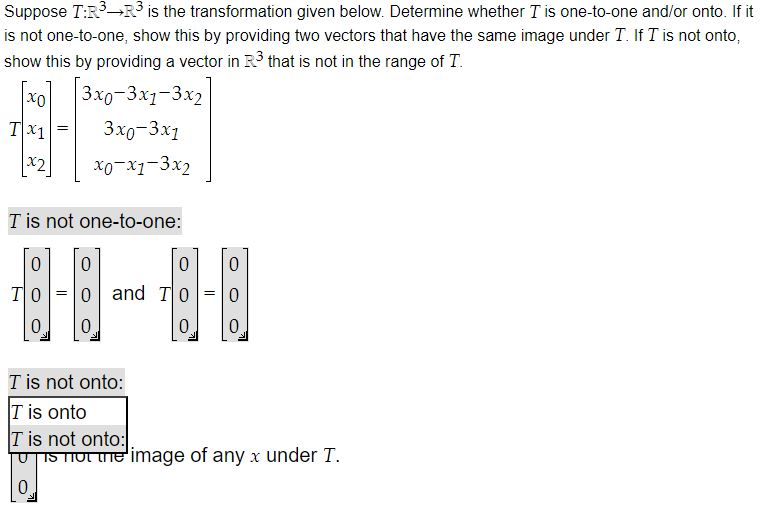Suppose T:R3-R3 is the transformation given below. Determine whether I is one-to-one and/or onto. If it is not one-to-one, show this by providing two vectors that have the same image under T. If T is not onto, show this by providing a vector in R3 that is not in the range of T. хо 3x0-3x4–3x2 3x0-3x1 x2 X0-X1-3x2 TX1 = T is not one-to-one: 0 0 0 0 TO = and TO 0 0 0 0 T is not onto: T is onto T is not onto: IS TIL Le image of any x under T. 0 U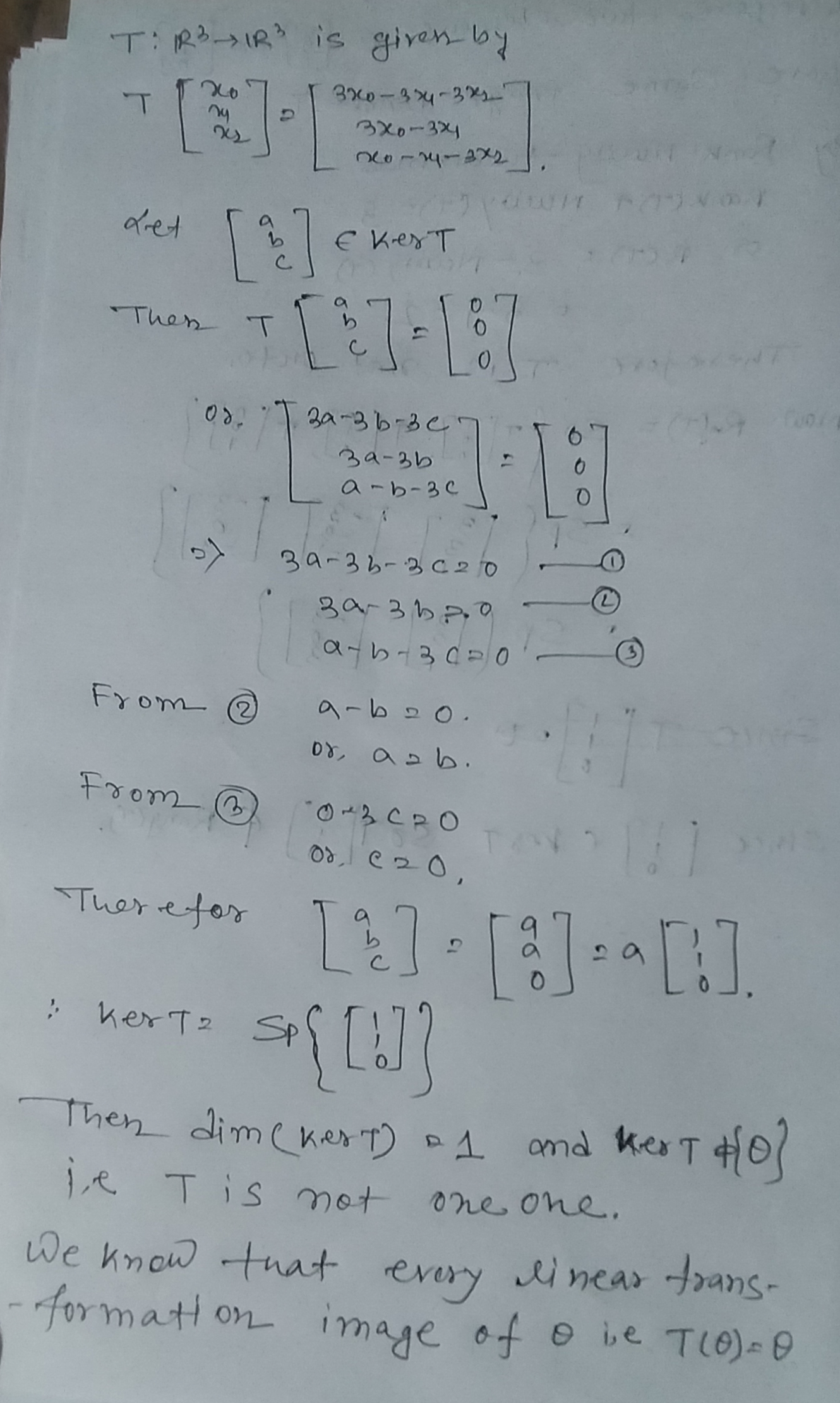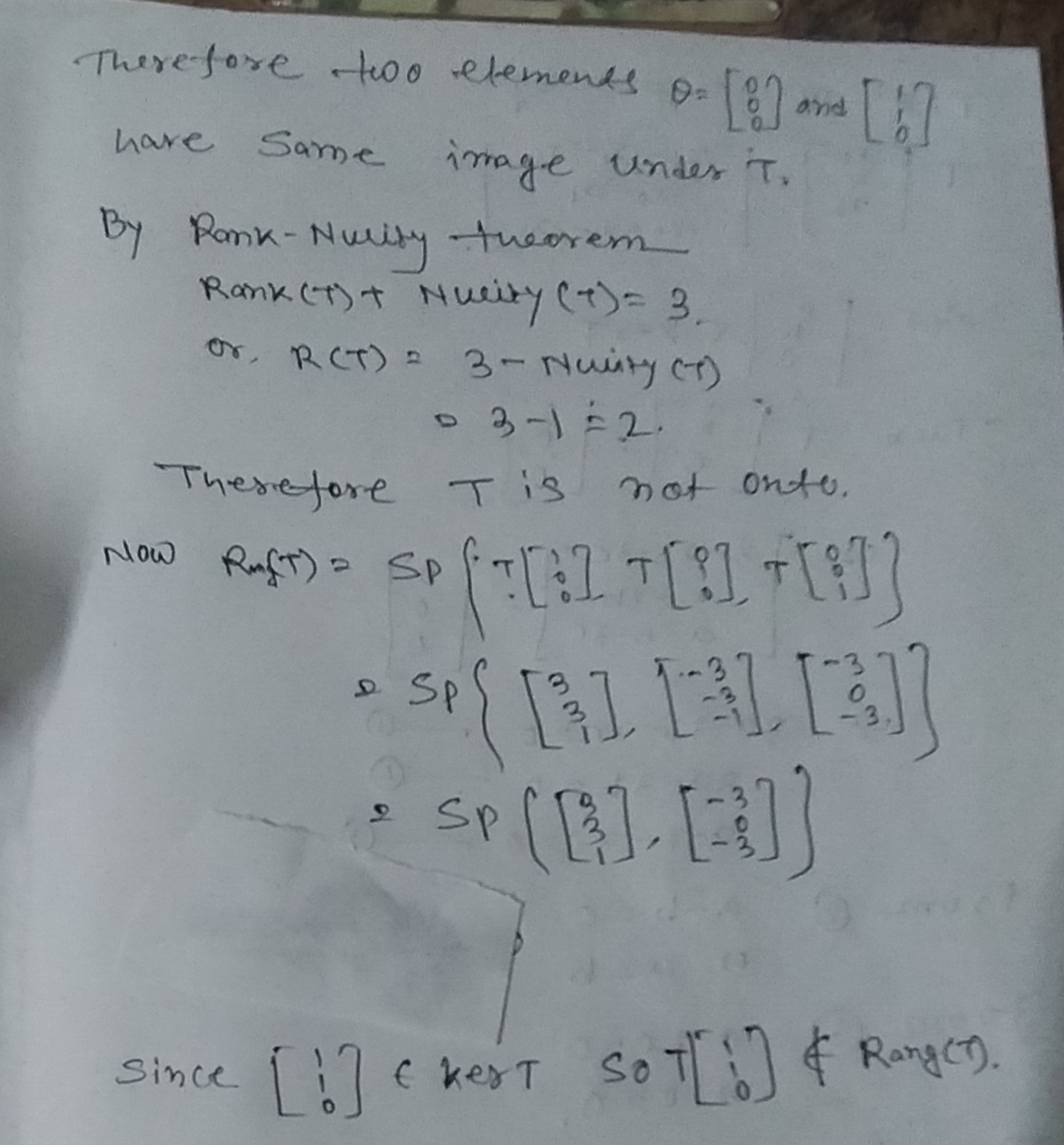#### Earn Coin

Coins can be redeemed for fabulous gifts.

Similar Homework Help Questions
• ### Suppose T:R4_R4 is the transformation given below. Determine whether T is one-to-one and/or onto. If it...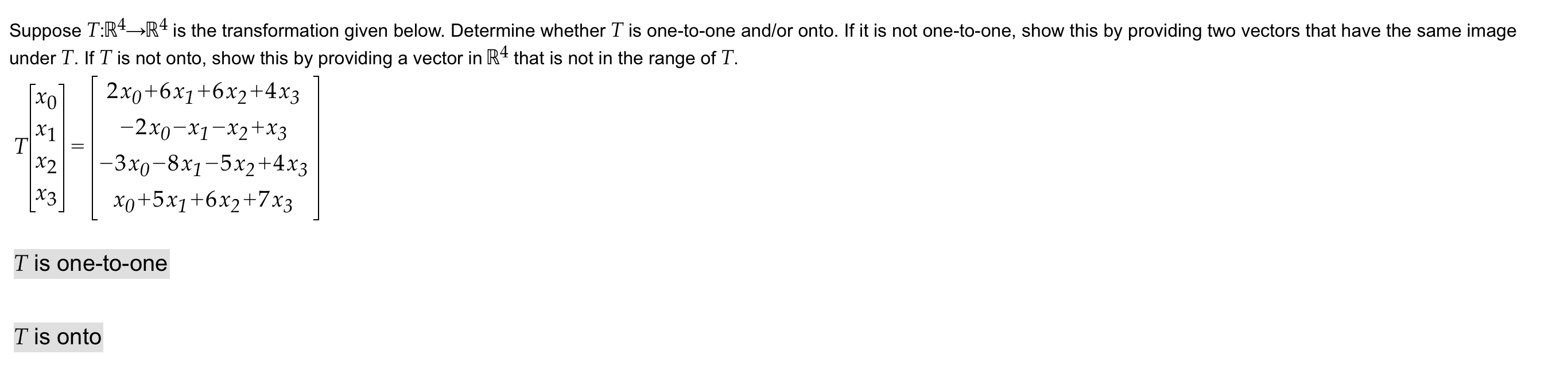Suppose T:R4_R4 is the transformation given below. Determine whether T is one-to-one and/or onto. If it is not one-to-one, show this by providing two vectors that have the same image under T. If T is not onto, show this by providing a vector in R4 that is not in the range of T. 2x0+6x1+6x2+4x3 -2x0–x1-x2 + x3 |-3x0-8x1-5x2+4x3 xo+5x1+6x2+7x3 2 Tis one-to-one Tis onto

• ### [E] Consider the linear transformation T: R3 → R3 given by: T(X1, X2, X3) = (x1...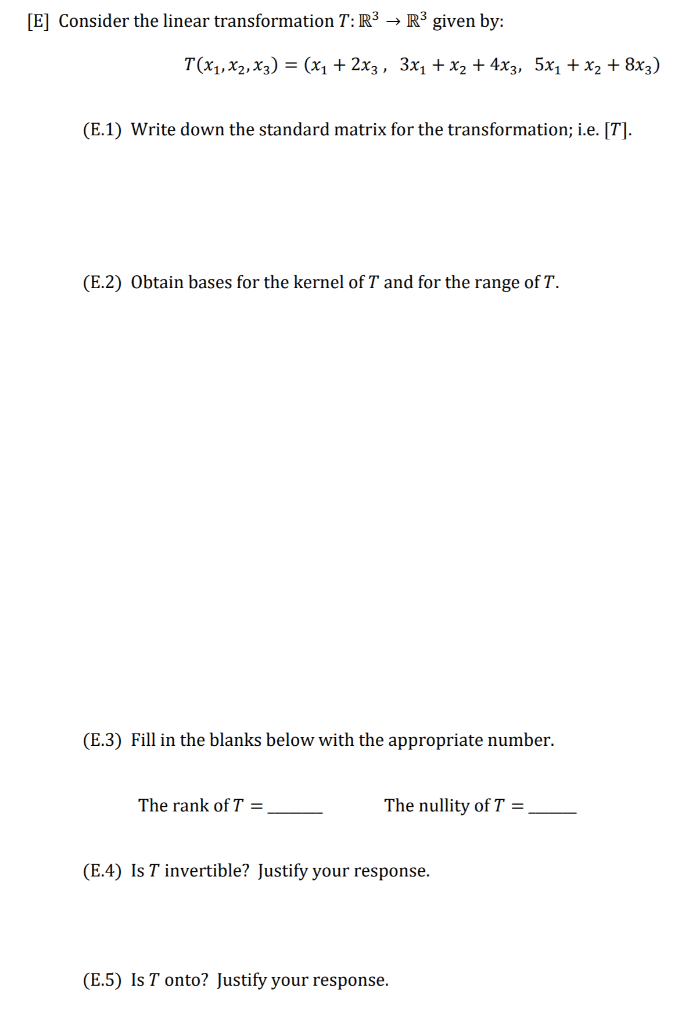[E] Consider the linear transformation T: R3 → R3 given by: T(X1, X2, X3) = (x1 + 2xz, 3x1 + x2 + 4x3, 5x1 + x2 + 8x3) (E.1) Write down the standard matrix for the transformation; i.e. [T]. (E.2) Obtain bases for the kernel of T and for the range of T. (E.3) Fill in the blanks below with the appropriate number. The rank of T = The nullity of T = (E.4) Is T invertible? Justify your response....

• ### Please show work Consider the following linear transformation T: RS → R3 where T(X1, X2, X3,...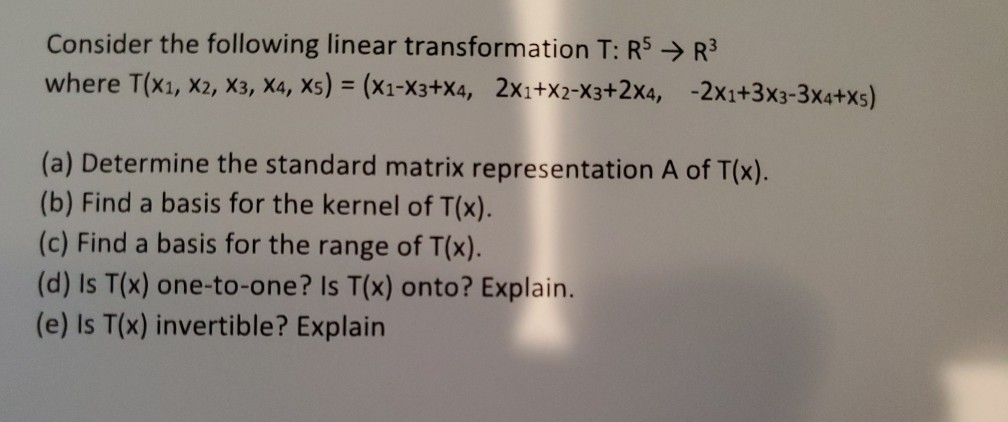Please show work Consider the following linear transformation T: RS → R3 where T(X1, X2, X3, X4, Xs) = (x1-X3+X4, 2x1+x2-X3+2x4, -2x1+3x3-3x4+xs) (a) Determine the standard matrix representation A of T(x). (b) Find a basis for the kernel of T(x). (c) Find a basis for the range of T(x). (d) Is T(x) one-to-one? Is T(x) onto? Explain. (e) Is T(x) invertible? Explain

• ### Consider the following linear transformation T: RS → R3 where T(X1, X2, X3, Xa, Xs) =...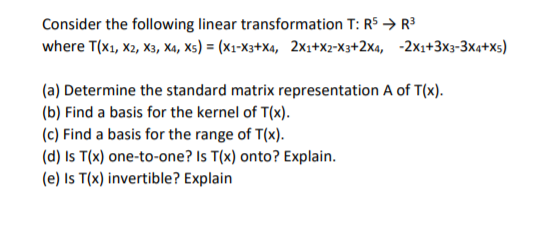Consider the following linear transformation T: RS → R3 where T(X1, X2, X3, Xa, Xs) = (x1-x3+Xa, 2x1+x2-x3+2x4, -2X2+3x3-3x4+xs) (a) Determine the standard matrix representation A of T(x). (b) Find a basis for the kernel of T(x). (c) Find a basis for the range of T(x). (d) Is T(x) one-to-one? Is T(x) onto? Explain. (e) Is T(x) invertible? Explain

• ### Consider the following linear transformation T: R5 → R3 where T(X1, X2, X3, X4, X5) =...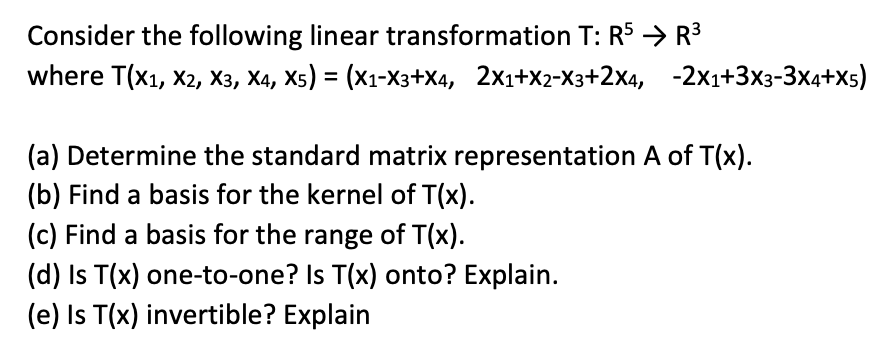Consider the following linear transformation T: R5 → R3 where T(X1, X2, X3, X4, X5) = (*1-X3+X4, 2X1+X2-X3+2x4, -2X1+3X3-3x4+x5) (a) Determine the standard matrix representation A of T(x). (b) Find a basis for the kernel of T(x). (c) Find a basis for the range of T(x). (d) Is T(x) one-to-one? Is T(x) onto? Explain. (e) Is T(x) invertible? Explain

• ### Question 8 [10 points] Suppose T: RM22 is a linear transformation whose action on a basis for R4 ...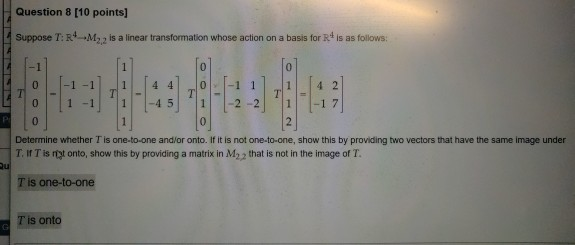Question 8 [10 points] Suppose T: RM22 is a linear transformation whose action on a basis for R4 is as follows -1 1 -11 4 4 0 1 1 0 1 -1 1 -45 1 2-2 1 -1 7 0 Determine whether T is one-to-one andlor onto. If it is not one-to-one, show this by providing two vectors that have the same image under T. If T is yt onto, show this by providing a matrix in M22 that is...

• ### Suppose T: P3-R is a linear transformation whose action on a basis for Pa is as...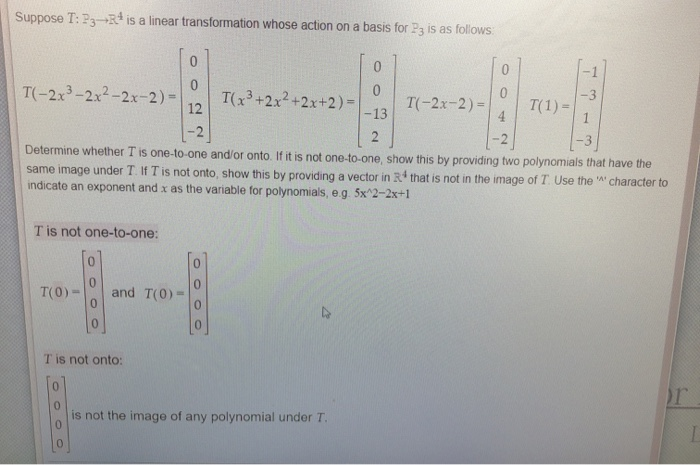Suppose T: P3-R is a linear transformation whose action on a basis for Pa is as follows 45 0 -3 0 0 T(-2x-2) T(-2x3-2x2-2x-2) T(x3+2x2+2x+2) = 12 T(1) 1 4 -13 |-2 -3 2 Determine whether T is one-to-one and/or onto. If it is not one-to-one, show this by providing two polynomials that have the same image under T If T is not onto, show this by providing a vector in R that is not in the image of T...

• ### Daoji and Drake are trying to find the determinant for the linear transformation T:R3 + R3...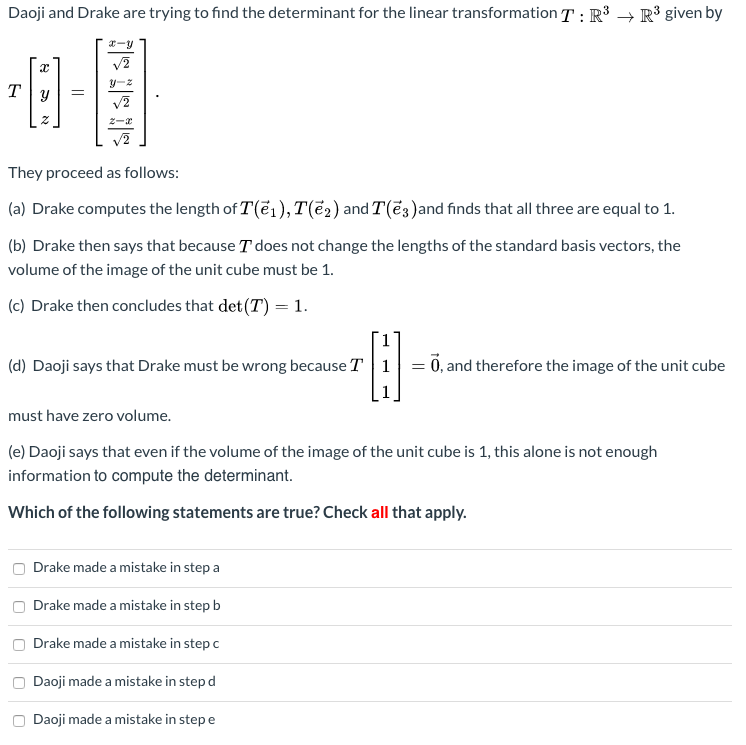Daoji and Drake are trying to find the determinant for the linear transformation T:R3 + R3 given by They proceed as follows: (a) Drake computes the length of T(@i), T(22) and T(3)and finds that all three are equal to 1. (b) Drake then says that because I does not change the lengths of the standard basis vectors, the volume of the image of the unit cube must be 1. (c) Drake then concludes that det(T) = 1. 17 (d) Daoji...

• ### Suppose T: R3–M2.2 is a linear transformation whose action on a basis for R3 is as...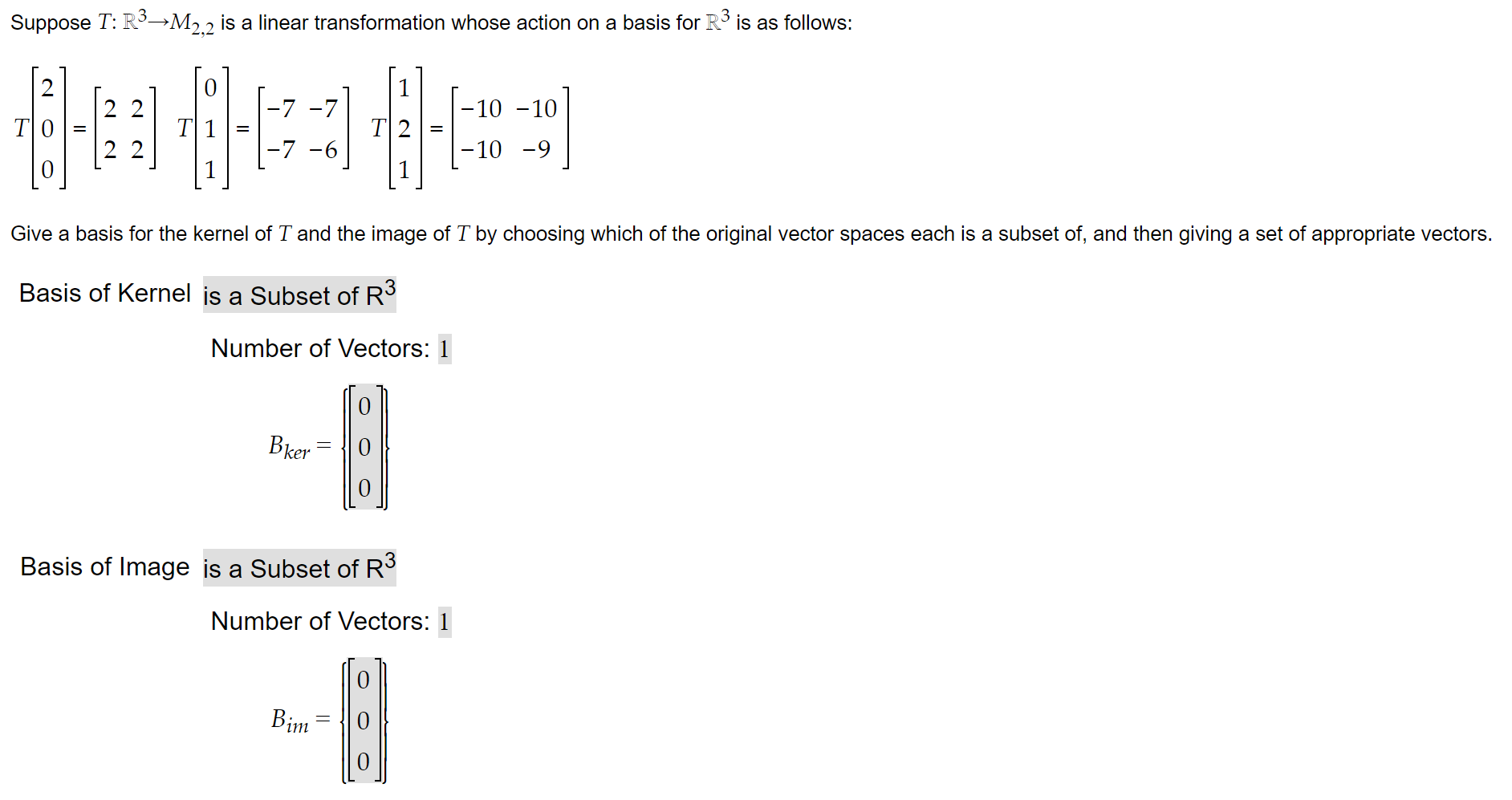Suppose T: R3–M2.2 is a linear transformation whose action on a basis for R3 is as follows: 0 -7 -7 -10 -10 T]01- T TI? 2 2 -7 -6 -10 -9 0 1 Give a basis for the kernel of T and the image of T by choosing which of the original vector spaces each is a subset of, and then giving a set of appropriate vectors. Basis of Kernel is a Subset of R3 Number of Vectors: 1 Bker...

• ### Problem 8. Define a transformation T : R2 + R3 by T(x1, x2) = (–2x1 –...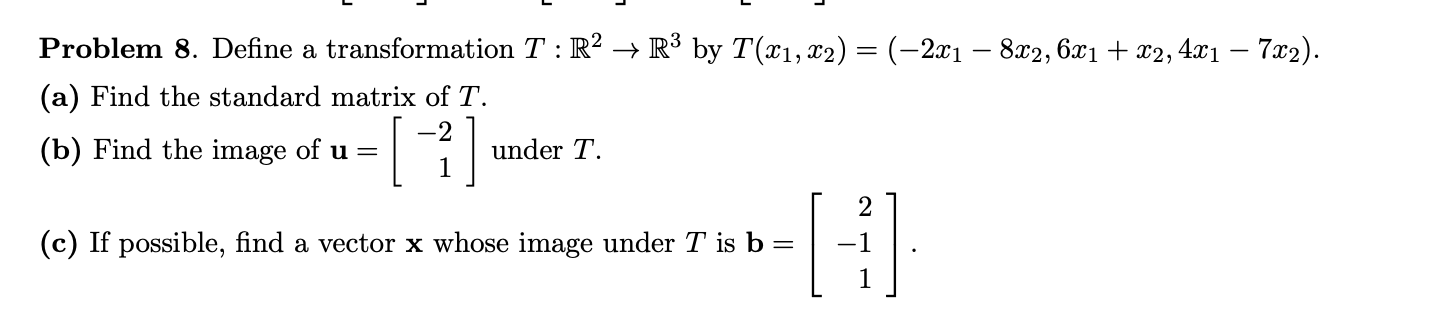Problem 8. Define a transformation T : R2 + R3 by T(x1, x2) = (–2x1 – 8x2,6x1 + x2, 4x1 – 7x2). (a) Find the standard matrix of T. (b) Find the image of u= under T. - 2  1 (c) If possible, find a vector x whose image under T is b = [ ། 2 -1